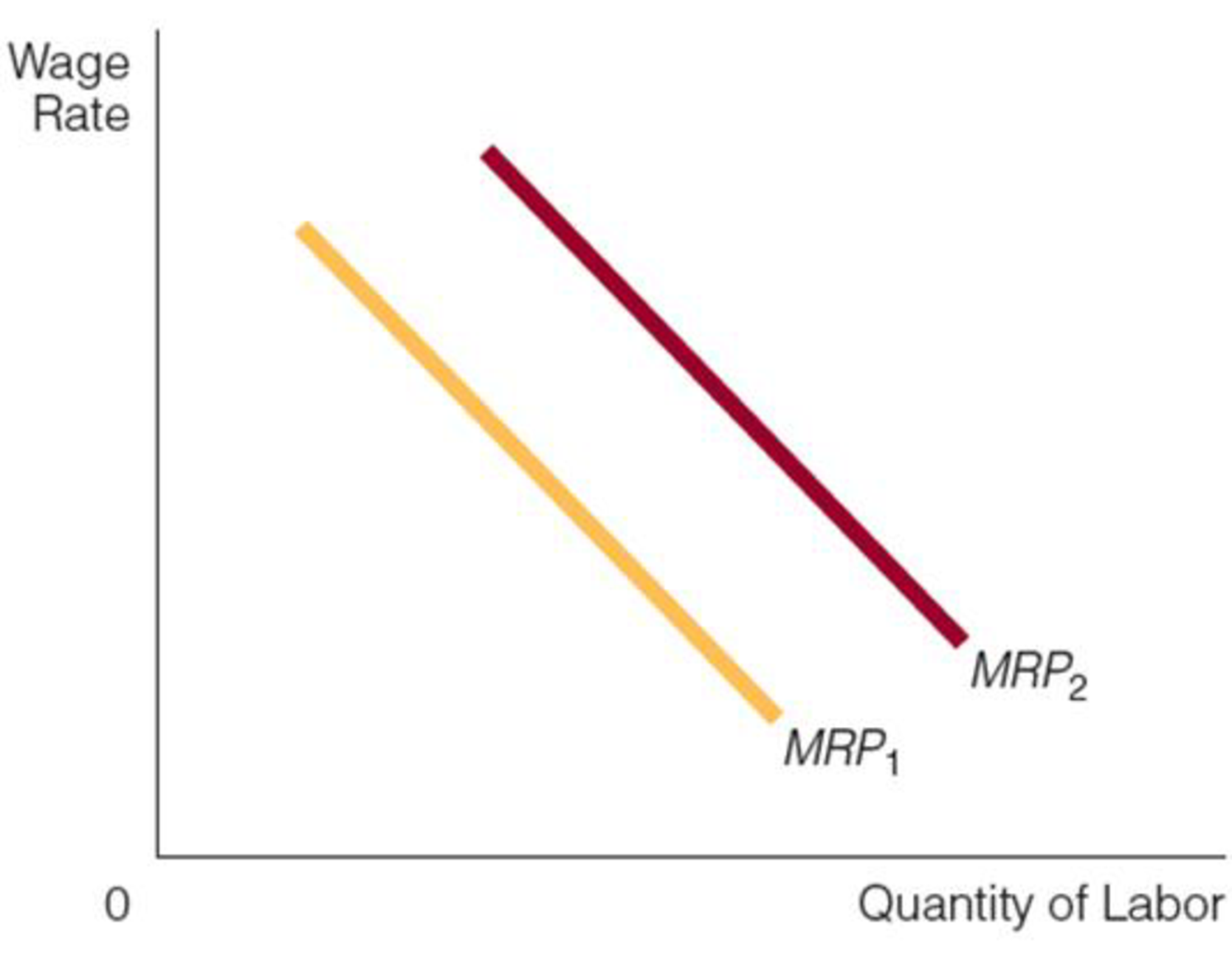Chapter 13, Problem 5WNG

Chapter
Section
Textbook Problem

Look at the two factor demand curves in the accompanying figure. Is the price of the product that labor goes to produce higher for MRP2 than for MRP1? Explain your answer.To determine

Compare the two demand curves.

Explanation

The figure shows, MRP1 and MRP2 curves.

In Figure (1), horizontal axis represents quantity of factor and vertical axis represents the wage rate. The MRP (Marginal Revenue Product) curve is the demand for factor curve, which can be calculated as MRP=MP×MR

Still sussing out bartleby?

Check out a sample textbook solution.

See a sample solution

The Solution to Your Study Problems

Bartleby provides explanations to thousands of textbook problems written by our experts, many with advanced degrees!

Get Started

Find more solutions based on key concepts